## Friday, 6 November 2009

### Problems printing pdfs in Fell

If you are having problems printing out pdfs in the Fell cluster you may wish to try a different cluster.

I have just received an email from ISS confirming there is a problem printing certain pdfs in the Fell cluster. As ISS says:

"Re following query, we have seen some problems with certain pdf files on the printer's in the fell cluster. We believe this is due to a firmware issue on these printers. Unfortunately the HP tool for upgrading large clusters of printers (web jetadmin) no longer runs on our Linux system, so we are for waiting for a Windows Server VM to be created before we can upgrade the FELL printers. We hope to have this completed in the near future, I will test your pdf again when this has been done and let you know the outcome."

So, if you run in to problems please go and try another cluster.....

## Monday, 2 November 2009

### CMB1005/7 BGM1001: Deriving Lineweaver-Burk Reciprocal Plot from Michaelis Menten Equation II

OK, well, here is another way Deriving Lineweaver-Burk Reciprocal Plot from Michaelis Menten Equation, this time starting from a different point (the other approach is here)...

The enzymatic reaction can be viewed as: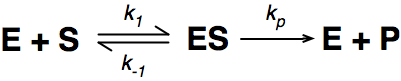Where E = enzyme; S = substrate; ES = Enzyme substrate complex; and P = product

The Michaelis-Menton Equation given in your lectures describing the reaction is:Where v = rate (initial velocity); Vmax = maximum velocity (100% of enzyme catalytic sites occupied); Km = Michaelis constant (concentration of substrate to achieve half Vmax); S = substrate concentration

In the lab we can change the substrate concentration (S) in a reaction and measure the rate (v). As you know, a plot of substrate concentration (S) against rate (v; initial velocity) gives a curve, which plateaus at Vmax, with Km the concentration at half-Vmax: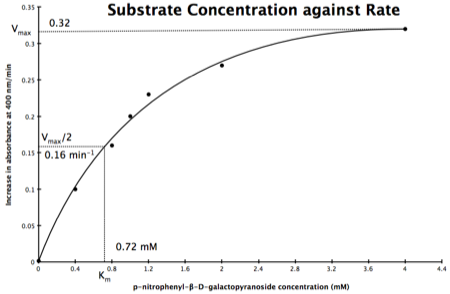Plot of Substrate Concentration against Initial Velocity (rate)

The direct measurement for Vmax can never be achieved in the lab as the concentration of the substrate needed would never be reached. Also, in the lab we would use a computer to calculate the values, and determine Vmax, and Km. However, you should be able to calculate Vmax, and Km yourself, just so you know the computer is right, and it is possible calculate these values from experimental data by using a linear plot and an equation derived from the Michaelis-Menton Equation.

As you know, the equation for a straight line is: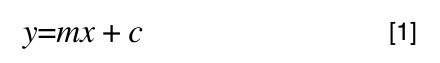Equation for a straight line, where m = the gradient and c = the intercept on the y-axis
So, the problem is, how do we get: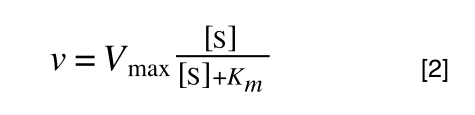to look like equation 1 so we can plot a straight line using the terms we can measure, i.e v and S? The answer is, we rearrange....

The first thing we need to do is invert equation 2 to get: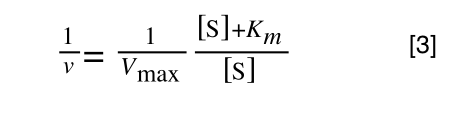If you didn’t understand that ‘mathematical move’ consider this: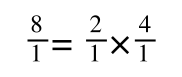The above is true. That is, 2 over 1 = 2, 4 over 1 = 4 and 2 times 4 is 8. If I simply invert (flip) all the parts: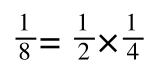It is also true.

Equation 3 is getting closer to what we want as we now have 1/v and this is our y in equation 1. All we need to do now is ‘extract’ x (which is our substrate concentration) from equation 3.

So, we have: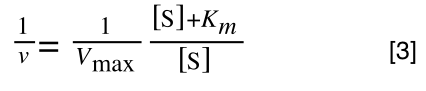which is the same as:If you consider the following it is true:

we can ‘separate terms’ on this and express it in several other ways: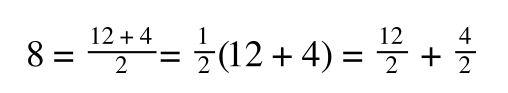That is, once we find a ‘common’ element (in the above example 1/2, and in equation 5 1 over [S]Vmax) we rearrange, so: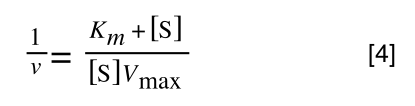extracting 1 over [S]Vmax in equation 4 we get:multiplying through with 1 over [S]Vmax gives: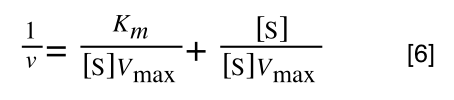As you can see in equation 6 we have two terms after the + that can cancel out, and our experimental variable (S) can be separated, so:

/div>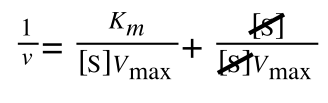so we get: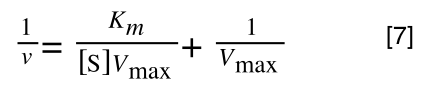If that bit of maths has lost you, consider this: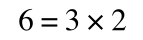if you divide both sides by 2 it is still correct: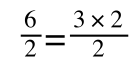However, on the righthand side the two 2s can be cancelled to give:which is still correct.

Finally, separating out 1/[S] from 7 gives: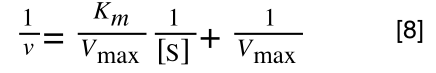Which when compare to equation 1: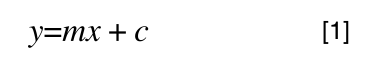It can be seen that:
• y = 1/v
• x = 1/[S]
• c, the y intercept = 1/Vmax
• m, the gradient = Km/Vmax
And, the intercept for the x axis, (i.e. when rate (y) = 0) is 1/-Km.

Hence, the final graph is: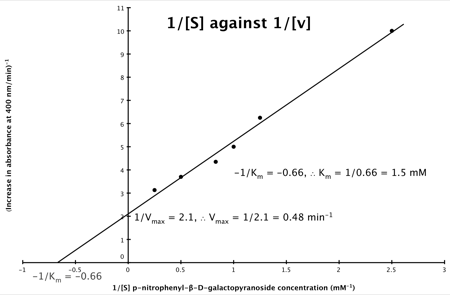If you would like to test your skills at performing enzyme kinetics calculations then you might like to look at: Maths4Biosciences.com.

## Sunday, 1 November 2009

### CMB1005/7 BGM1001: Deriving Lineweaver-Burk Reciprocal Plot from Michaelis Menten Equation I

After the a recent seminar a number of students have asked how to derive the Lineweaver-Burk Reciprocal Plot equation from the Michaelis Menten equation... so, here goes.

The enzymatic reaction can be viewed as:Where E = enzyme; S = substrate; ES = Enzyme substrate complex; and P = product

The Michaelis-Menton Equation describing the reaction is: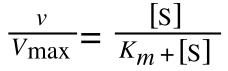Where v = rate (initial velocity); Vmax = maximum velocity (100% of enzyme catalytic sites occupied); Km = Michaelis constant (concentration of substrate to achieve half Vmax); S = substrate concentration

Now, if the equation you are starting with does not look like the one above then have a look at another post. Both Dr Bevitt and Prof Lakey both start the rearrangement of the equation from the point shown in the other post (apparently I do it the 'old fashioned way'!)

In the lab we can change the substrate concentration (S) in a reaction and measure the rate (v). As you know, a plot of substrate concentration (S) against rate (v; initial velocity) gives a curve, which plateaus at Vmax, with Km the concentration at half-Vmax:Plot of Substrate Concentration against Initial Velocity (rate)

The direct measurement for Vmax can never be achieved in the lab as the concentration of the substrate needed would never be reached. Also, in the lab we would use a computer to calculate the values, and determine Vmax, and Km. However, you should be able to calculate Vmax, and Km yourself, just so you know the computer is right, and it is possible calculate these values from experimental data by using a linear plot and an equation derived from the Michaelis-Menton Equation.

As you know, the equation for a straight line is:Equation for a straight line, where m = the gradient and c = the intercept on the y-axis

So, the problem is, how do we get: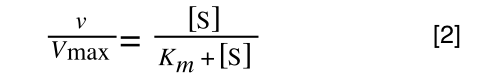to look like equation 1? The answer is, we rearrange....

Rearranging

We need to 'extract' our substrate concentration S and our rate v so we can plot them on a straight line graph. Basically we need to get equation 2 to look like equation 1.

The equation for a straight line is:And our Michaelis-Menton Equation:So, the first thing we need to do is invert equation 2 to get: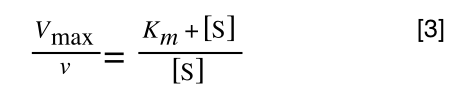If you didn’t understand that ‘mathematical move’ consider this:The above is true. That is, 2 over 1 = 2, 4 over 1 = 4 and 2 times 4 is 8. If I simply invert (flip) all the parts:It is also true.

Equation 3 is getting closer to what we want. However, the Vmax over v is a problem as we don’t know Vmax and can only measure v and S in the lab. Therefore, we need to separate out the terms we can measure so we can have x and y as in equation 1.

To ‘remove’ the Vmax from the lefthand side we need to divide both sides by Vmax: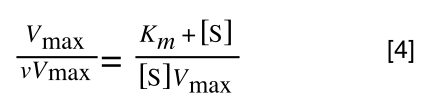Note the new Vmax term on both sides of the equation - compare to equation 3

As we now have Vmax over v multiplied by Vmax we can cancel out both Vmax: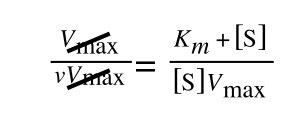to give: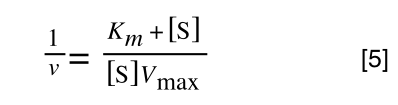If that bit of maths has lost you, consider this:if you divide both sides by 2 it is still correct:However, on the righthand side the two 2s can be cancelled to give: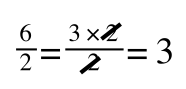which is still correct.

In equation 5 we now have 1/v and this is our y in equation 1. All we need to do now is ‘extract’ x (which is our substrate concentration) from equation 5.

If you consider the following it is true:we can ‘separate terms’ on this and express it in several other ways:That is, once we find a ‘common’ element (in the above example 1/2, and in equation 5 1 over [S]Vmax) we rearrange, so: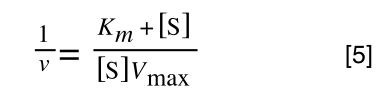extracting 1 over [S]Vmax we get:multiplying through with 1 over [S]Vmax gives:As you can see in equation 7 we have two terms after the + that can cancel out, and our experimental variable (S) can be separated, so: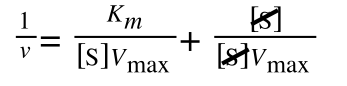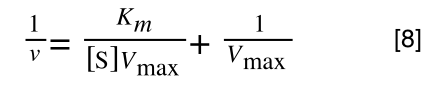Finally, separating out 1/[S] gives: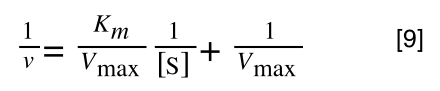Which when compare to equation 1:It can be seen that:
• y = 1/v
• x = 1/[S]
• c, the y intercept = 1/Vmax
• m, the gradient = Km/Vmax
And, the intercept for the x axis, (i.e. when rate (y) = 0) is 1/-Km.

Hence, the final graph is: